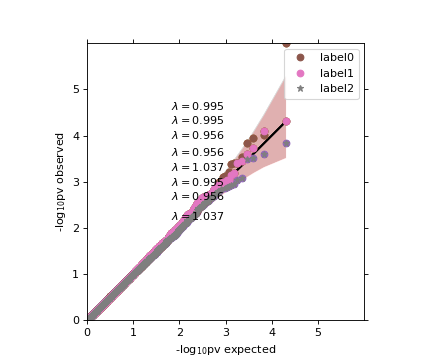# Limix-plot’s documentation¶

Plotting library for genetics.

# Consensus curve¶

class `limix_plot.``ConsensusCurve`[source]

Consolidate multiple curves in a single one.

Example

```>>> import limix_plot as lp
>>> from numpy import sort
>>> from numpy.random import RandomState
>>>
>>> random = RandomState(1)
>>>
>>> n0 = 30
>>> x0 = sort(random.rand(n0))
>>> y0 = 10 * x0 + random.rand(n0)
>>>
>>> n1 = 20
>>> x1 = sort(random.rand(n1))
>>> y1 = 10 * x1 + random.rand(n1)
>>>
>>> cc = lp.ConsensusCurve()
>>>
>>> (x, ybottom, y, ytop) = cc.consensus()
>>> ax = lp.get_pyplot().gca()
>>> _ = ax.plot(x, y)
>>> _ = ax.fill_between(x, ybottom, ytop, lw=0, edgecolor='None',
...                     facecolor='blue',  alpha=0.25, interpolate=True)
>>> lp.show()
```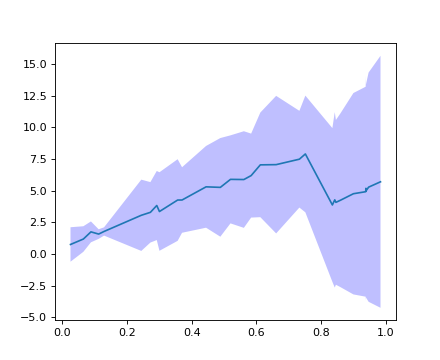`add`(x, y)[source]

Parameters: x (array_like) – x-coordinate values. y (array_like) – y-coordinate values.
`consensus`(std_dev=3.0)[source]

Return a consensus curve.

Parameters: std_dev (float) – Confidence band width. Defaults to `3`. x (array_like) – x-coordinate values. ybottom (array_like) – y-coordinate values of the lower part of the confidence band. y (array_like) – y-coordinate values of the mean of the confidence band. ytop (array_like) – y-coordinate values of the upper part of the confidence band.

# Heatmap plot for kinship matrix¶

`limix_plot.``kinship`(K, nclusters=1, img_kws=None, ax=None)[source]

Plot heatmap of a kinship matrix.

Parameters: K (2d-array) – Kinship matrix. nclusters (int, str, optional) – Number of blocks to be seen from the heatmap. It defaults to `1`, which means that no ordering is performed. Pass `"auto"` to automatically determine the number of clusters. Pass an integer to select the number of clusters. img_kws (dict, optional) – Keyword arguments forwarded to the matplotlib pcolormesh function. ax (matplotlib Axes, optional) – The target handle for this figure. If `None`, the current axes is set.

Example

```>>> import limix_plot as lp
>>>
>>> lp.kinship(K)
```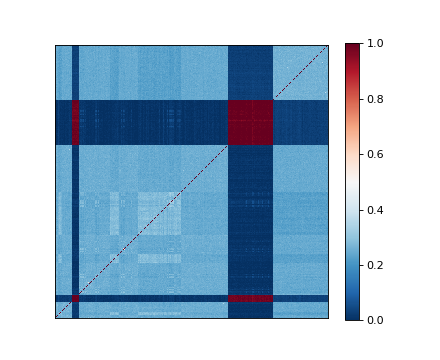# Manhattan plot¶

`limix_plot.``manhattan`(data, colora='#5689AC', colorb='#21334F', pts_kws=None, ax=None)[source]

Produce a manhattan plot.

Parameters: data (DataFrame, dict) – DataFrame containing the chromosome, base-pair positions, and p-values. colora (matplotlib color) – Points color of the first group. colorb (matplotlib color) – Points color of the second group. pts_kws (dict, optional) – Keyword arguments forwarded to the matplotlib function used for plotting the points. ax (matplotlib Axes, optional) – The target handle for this figure. If `None`, the current axes is set.

Example

```>>> import limix_plot as lp
>>> from numpy import log10
>>>
>>> df = df.rename(columns={"chr": "chrom"})
chrom     pos       pv
234    10  224239  0.00887
239    10  229681  0.00848
253    10  240788  0.00721
258    10  246933  0.00568
266    10  255222  0.00593
>>> lp.manhattan(df)
>>> plt = lp.get_pyplot()
>>> _ = plt.axhline(-log10(1e-7), color='red')
>>> _ = plt.ylim(2, plt.ylim())
```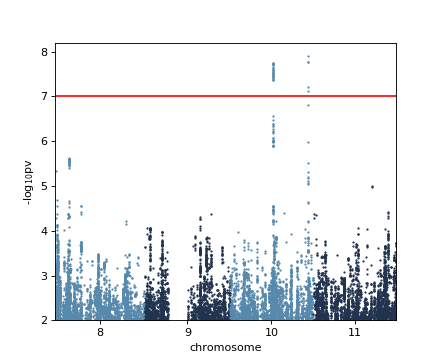# Normal plot¶

`limix_plot.``normal`(x, bins=20, nstd=2, ax=None)[source]

Plot a fit of a normal distribution to the data in x.

Parameters: x (array_like) – Values to be fitted. bins (int, optional) – Number of histogram bins. Defaults to `20`. nstd (float, optional) – Standard deviation multiplier for drawing a dashed line. ax (matplotlib Axes, optional) – The target handle for this figure. If `None`, the current axes is set.

Example

```>>> from numpy.random import RandomState
>>> import limix_plot as lp
>>>
>>> random = RandomState(10)
>>> x = random.randn(100)
>>> lp.normal(x)
```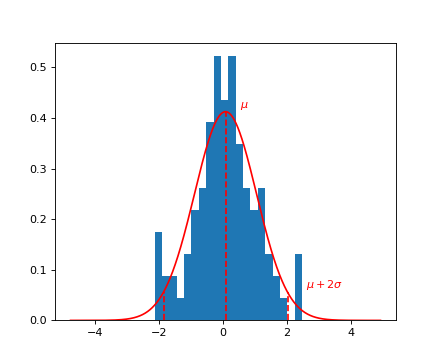# PCA plot¶

`limix_plot.``pca`(X, pts_kws=None, ax=None)[source]

Plot the first two principal components of a design matrix.

Parameters: X (array_like) – Design matrix: samples by features. pts_kws (dict, optional) – Keyword arguments forwarded to the matplotlib pcolormesh function. ax (matplotlib Axes, optional) – The target handle for this figure. If `None`, the current axes is set.

Example

```>>> import limix_plot as lp
>>> from numpy.random import RandomState
>>>
>>> random = RandomState(0)
>>> X = random.randn(30, 10)
>>> lp.pca(X)
```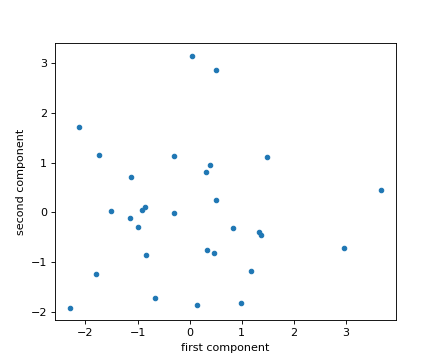# Plot an image¶

`limix_plot.``image`(file, ax=None)[source]

Show an image.

Parameters: file (file-like object, string, or pathlib.Path) – The file to read. ax (matplotlib Axes, optional) – The target handle for this figure. If `None`, the current axes is set.

Example

```>>> import limix_plot as lp
>>>
>>> lp.image(file)
>>> file.close()
```# Power plot¶

`limix_plot.``power`(pv, label=None, alphas=None, pts_kws=None, ax=None)[source]

Plot number of hits across significance levels.

Parameters: pv (array_like) – P-values. label (string, optional) – Legend label for the relevent component of the plot. alphas (array_like, optional) – Significance thresholds for which the number of hits is defined. Defaults to `numpy.linspace(0.01, 0.5, 500)`. pts_kws (dict, optional) – Keyword arguments forwarded to the matplotlib function used for plotting the points. ax (matplotlib Axes, optional) – The target handle for this figure. If `None`, the current axes is set.

Example

```>>> import limix_plot as lp
>>> from numpy.random import RandomState
>>>
>>> random = RandomState(1)
>>> nsnps = 10000
>>>
>>> pv0 = list(random.rand(nsnps))
>>> pv1 = list(0.7 * random.rand(nsnps))
>>>
>>> lp.power(pv0, label='label0')
>>> lp.power(pv1, label='label1')
>>> _ = lp.get_pyplot().legend(loc='best')
```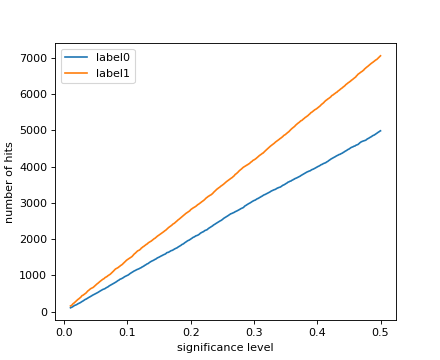# Quantile-quantile plot¶

`limix_plot.``qqplot`(a, label=None, alpha=0.05, cutoff=0.1, line=True, pts_kws=None, band_kws=None, ax=None, show_lambda=True)[source]

Quantile-Quantile plot of observed p-values versus theoretical ones.

Parameters: a (Series, 1d-array, list) – Observed p-values. label (string, optional) – Legend label for the relevent component of the plot. alpha (float, optional) – Significance level defining the confidfence interval. Set to `None` to disable plotting. Defaults to `0.05`. cutoff (float, optional) – P-values higher than cutoff will not be plotted. Defaults to `0.1`. line (bool, optional) – Whether or not plot a straight line. Defaults to `True`. pts_kws (dict, optional) – Keyword arguments forwarded to the matplotlib function used for plotting the points. band_kws (dict, optional) – Keyword arguments forwarded to the fill_between function used for plotting the confidence band. ax (matplotlib Axes, optional) – The target handle for this figure. If `None`, the current axes is set.

Example

```>>> import limix_plot as lp
>>> from numpy.random import RandomState
>>>
>>> random = RandomState(1)
>>>
>>> pv0 = random.rand(10000)
>>> pv0 = 1e-6
>>>
>>> pv1 = random.rand(10000)
>>> pv2 = random.rand(10000)
>>>
>>> lp.qqplot(pv0)
>>>
>>> lp.qqplot(pv0)
>>> lp.qqplot(pv1, line=False, alpha=None)
>>>
>>> lp.qqplot(pv1)
>>> lp.qqplot(pv2, line=False, alpha=None)
>>> lp.box_aspect()
>>>
```
```>>> lp.qqplot(pv0, label='label0', band_kws=dict(color='#EE0000',
...           alpha=0.2));
>>> lp.qqplot(pv1, label='label1', line=False, alpha=None);
>>> lp.qqplot(pv2, label='label2', line=False,
...           alpha=None, pts_kws=dict(marker='*'));
>>> _ = lp.get_pyplot().legend()
```